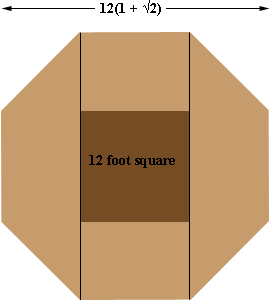SEARCH HOMEMath Central Quandaries & QueriesQuestion from Tony: I am building a pen for my horse. I am going to use 12' panels in the shape of an octagon. How many feet will he have from side to side using 12' panels. I am not a math genius so be simple please. I have asked a doctor, lawyer, a math professor and a chemistry major and no one can answer my question. Thanks, TonyTony, we have two responses for you. Penny thought you were going to cut 4 corners from a 12 foot panel and RD thought you were going to start with a 12 foot panel and build out to form the octagon. Harley

Hi Tony,

We received a very similar question a while ago from Freddie. He however was using a 48 inch square where yours is 12 feet which is 12 × 12 = 144 inches. Look at the diagram in my response to Freddie. I showed that y = 48/(√2 + 2). In your case that's y = 144/(√2 + 2) = 42.1766 inches which is approximately 42 3/16 inches.

I hope this helps,
Penny

Hi Tony,

The octagon can be divided into three parts: a central rectangle and two trapezoids:The trapezoids will each be 12/sqrt(2) feet wide and the rectangle will be 12 feet wide, for a total of 12(1 + sqrt(2)) feet [you can see how to scale this for other panel sizes.] This works out as almost exactly 29 feet, side to side.

If you measure from corner to corner, it will be slightly larger (by a factor of sec(22.5 degrees)) and the diameter will be about 31 feet 4 inches.

RDMath Central is supported by the University of Regina and The Pacific Institute for the Mathematical Sciences.